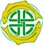﻿ Non-metric Multi-Dimensional ScalingNon-metric Multi-Dimensional Scaling

Multi-Dimensional Scaling (MDS) is a technique for expressing the similarities between different objects in a small number of dimensions. Hopefully, this allows a complex set of inter-relationships to be summarised in a simple figure. The method attempts to place the most similar objects (samples) closest together. The starting point for the calculations is a similarity or dissimilarity matrix between all the sites or quadrats. These can be non-metric distance measures for which the relationships between the sites/objects/samples (columns) cannot be plotted in a Euclidean space. The aim of Non-metric MDS is to find a set of metric coordinates for the sites which most closely approximates their non-metric distances.

The basic MDS algorithm is as follows:

 1 Calculate the similarity or dissimilarity between sites.
 2 Assign to each site a set of coordinates in p-dimensional space. These coordinates can be either chosen at random or chosen using Principal Coordinates Analysis (note, this is not the same as a Principal Component Analysis). The value of p is chosen by the user.
 3 Compute the Euclidean distance between these sites using the starting coordinates.
 4 Compare the original dissimilarity between the sites with these Euclidean distances by calculating a stress function. The smaller the stress function, the closer the correspondence.
 5 Adjust the positions so as to reduce the stress.
 6 Repeat 2 to 4 until the stress is minimised or the maximum number of iterations is reached.

CAP uses Kruskals's least squares monotonic transformation to minimise the stress (see Kruskal, 1964; Kruskal & Wish, 1977). The program is designed to find an optimal two-dimensional representation of the data. It can happen that no useful two-dimensional representation can be produced. While it is possible to produce anything up to a six-dimensional solution, in practice this is of little use, as it cannot be displayed. When requested, CAP lists solutions for 3 or more dimensions, but does not plot them.

From the ordination drop-down menu select Non-metric MDS. The setup for Non-metric MDS is displayed. This offers a series of options that are described in NMDS Starting Configuration. The program can usually be run with the default values.

Output from Non-metric MDS is presented under a number of tabbed components that can each be viewed by clicking on the tab. These are described in turn below:

MDS setup

MDS plots

Sample coordinates

Stress